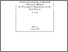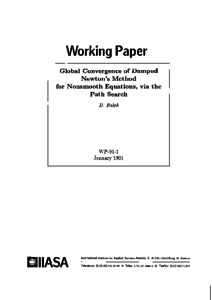# Global Convergence of Damped Newton's Method for Nonsmooth Equations, via the Path Search

Ralph, D. (1991). Global Convergence of Damped Newton's Method for Nonsmooth Equations, via the Path Search. IIASA Working Paper. IIASA, Laxenburg, Austria: WP-91-001Preview
Text
WP-91-001.pdf

## Abstract

A natural damping of Newton's method for nonsmooth equations is presented. This damping, via the path search instead of the traditional line search, enlarges the domain of convergence of Newton's method and therefore is said to be globally convergent. Convergence behavior is like that of line search damped Newton's method for smooth equations, including Q-quadratic convergence rates under appropriate conditions.

Applications of the path search include damping Robinson-Newton's method for nonsmooth normal equations corresponding to nonlinear complementarity problems and variational inequalities, hence damping both Wilson's method (sequential quadratic programming) for nonlinear programming and Josephy-Newton's method for generalized equations.

Computational examples from nonlinear programming are given.

Item Type: Monograph (IIASA Working Paper) Adaption and Optimization (ADO)System and Decision Sciences - Core (SDS) IIASA Import 15 Jan 2016 02:01 27 Aug 2021 17:14 http://pure.iiasa.ac.at/3562View Item